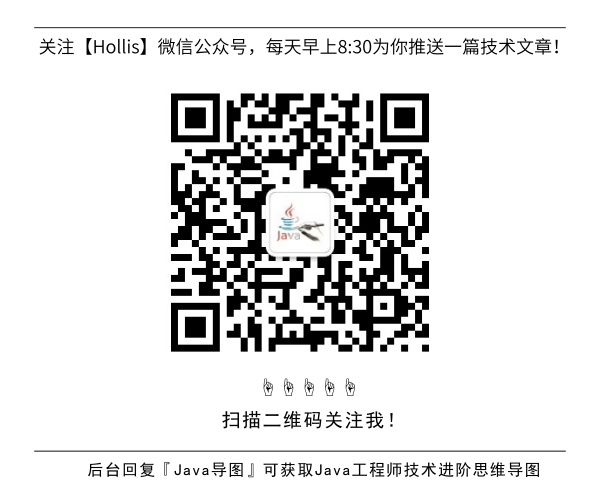# [译]Java中整型的缓存机制

Hollis的新书《深入理解Java核心技术》出版了

``````package com.javapapers.java;

public class JavaIntegerCache {
public static void main(String... strings) {

Integer integer1 = 3;
Integer integer2 = 3;

if (integer1 == integer2)
System.out.println("integer1 == integer2");
else
System.out.println("integer1 != integer2");

Integer integer3 = 300;
Integer integer4 = 300;

if (integer3 == integer4)
System.out.println("integer3 == integer4");
else
System.out.println("integer3 != integer4");

}
}
``````

``````integer1 == integer2
integer3 != integer4
``````

## Java中Integer的缓存实现

Java的编译器把基本数据类型自动转换成封装类对象的过程叫做`自动装箱`，相当于使用`valueOf`方法：

``````Integer a = 10; //this is autoboxing
Integer b = Integer.valueOf(10); //under the hood
``````

``````/**
* Returns an {@code Integer} instance representing the specified
* {@code int} value.  If a new {@code Integer} instance is not
* required, this method should generally be used in preference to
* the constructor {@link #Integer(int)}, as this method is likely
* to yield significantly better space and time performance by
* caching frequently requested values.
*
* This method will always cache values in the range -128 to 127,
* inclusive, and may cache other values outside of this range.
*
* @param  i an {@code int} value.
* @return an {@code Integer} instance representing {@code i}.
* @since  1.5
*/
public static Integer valueOf(int i) {
if (i >= IntegerCache.low && i <= IntegerCache.high)
return IntegerCache.cache[i + (-IntegerCache.low)];
return new Integer(i);
}
``````

## IntegerCache Class

IntegerCache是Integer类中定义的一个`private static`的内部类。接下来看看他的定义。

``````  /**
* Cache to support the object identity semantics of autoboxing for values between
* -128 and 127 (inclusive) as required by JLS.
*
* The cache is initialized on first usage.  The size of the cache
* may be controlled by the {@code -XX:AutoBoxCacheMax=} option.
* During VM initialization, java.lang.Integer.IntegerCache.high property
* may be set and saved in the private system properties in the
* sun.misc.VM class.
*/

private static class IntegerCache {
static final int low = -128;
static final int high;
static final Integer cache[];

static {
// high value may be configured by property
int h = 127;
String integerCacheHighPropValue =
sun.misc.VM.getSavedProperty("java.lang.Integer.IntegerCache.high");
if (integerCacheHighPropValue != null) {
try {
int i = parseInt(integerCacheHighPropValue);
i = Math.max(i, 127);
// Maximum array size is Integer.MAX_VALUE
h = Math.min(i, Integer.MAX_VALUE - (-low) -1);
} catch( NumberFormatException nfe) {
// If the property cannot be parsed into an int, ignore it.
}
}
high = h;

cache = new Integer[(high - low) + 1];
int j = low;
for(int k = 0; k < cache.length; k++)
cache[k] = new Integer(j++);

// range [-128, 127] must be interned (JLS7 5.1.7)
assert IntegerCache.high >= 127;
}

private IntegerCache() {}
}
``````

## Java语言规范中的缓存行为

Boxing Conversion部分的Java语言规范(JLS)规定如下：

-128至127之间的整数(§3.10.1)

true 和 false的布尔值 (§3.10.3)

‘\u0000’至 ‘\u007f’之间的字符(§3.10.4)

## 其他缓存的对象

`Byte`, `Short`, `Long`有固定范围: -128 到 127。对于`Character`, 范围是 0 到 127。除了`Integer`以外，这个范围都不能改变。

(全文完)## HollisChuang's Blog

• 微信咨询
• 去评论
• 回顶
###### 回顶部